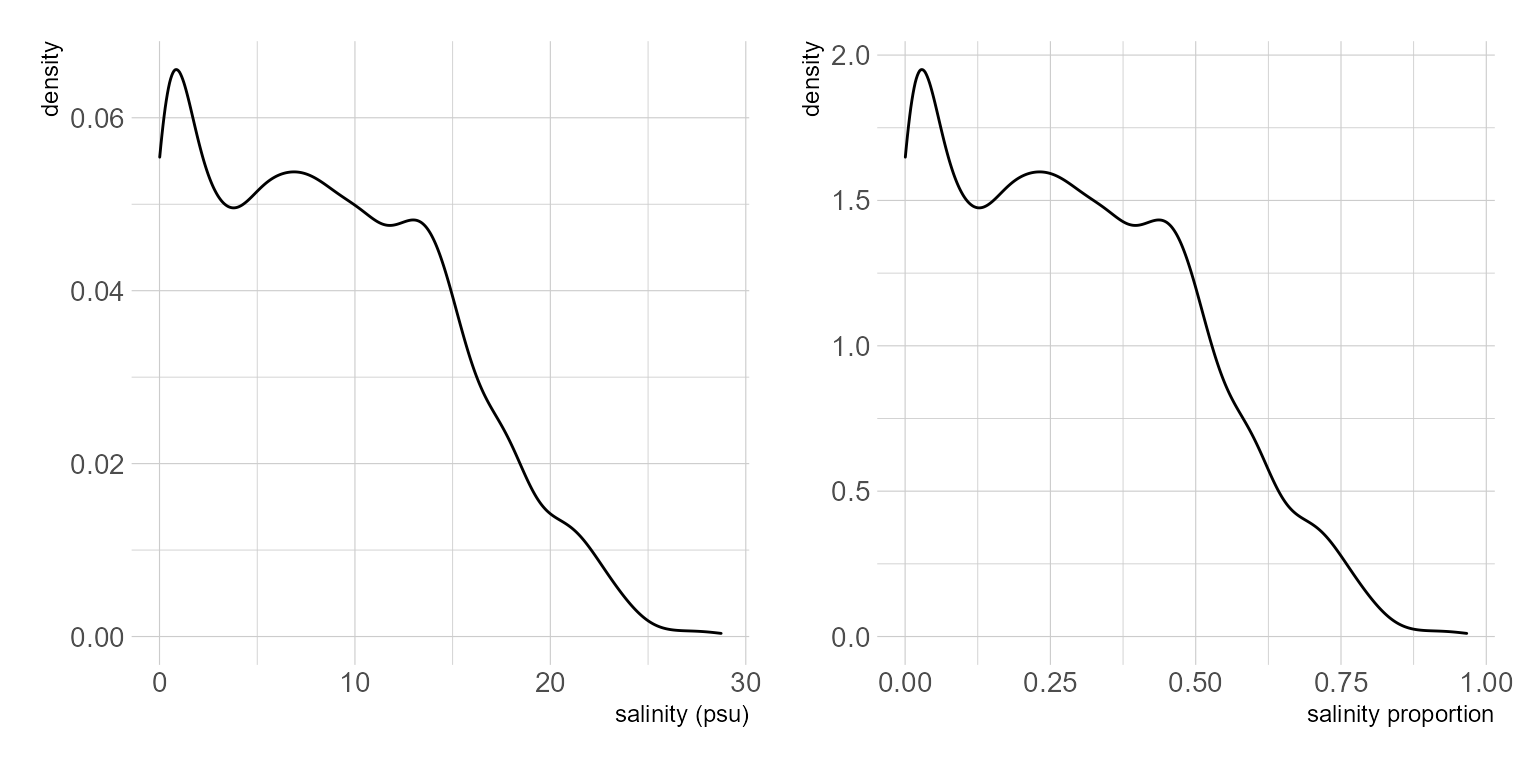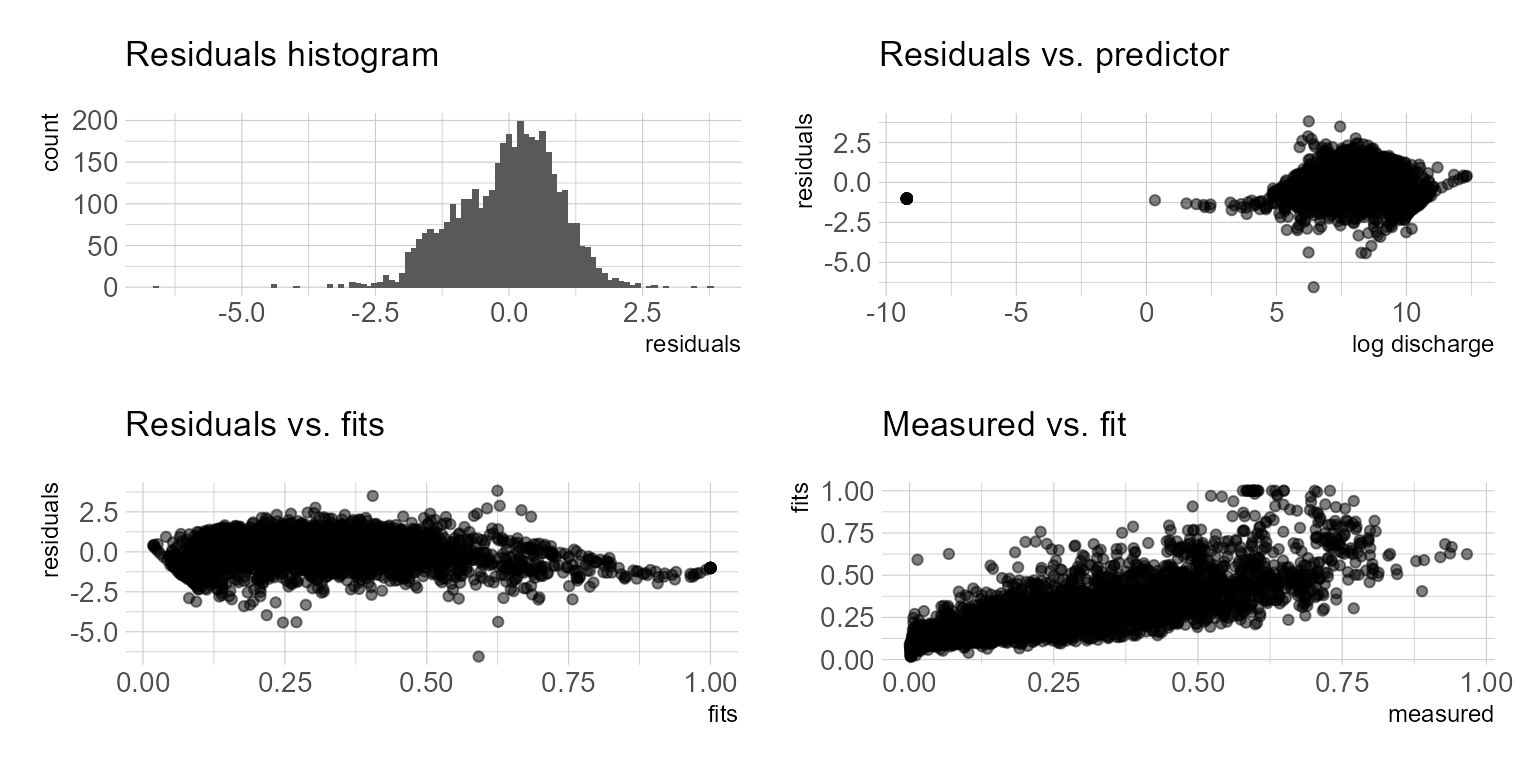# Predicting estuarine salinity using simple statistical models part 2

Part one of some statistical approaches for estimating estuarine salinity using freshwater inflow.

Michael Schramm https://michaelpaulschramm.com (Texas Water Resources Institute)https://twri.tamu.edu
2020-09-09

In the previous post I used nonlinear least squares to fit a logistic function to some salinity data. Here I explore using beta regression. Beta regression is appropriate for inherently proportional data (as opposed to proportion of success/failure or rates with shifting denominators, both covered by logistic and Poisson regression). provide a great flow chart for determining the type of model to use with proportional data. Of course, the salinity data we use comes reported in practical salinity units (psu). We can make an assumption that the estuary has some maximum salinity and ceate a response variable that is in a proportion unit. More on that in a bit. I will be using the same salinity and flow dataset loaded in the previous post.

Beta regression is appropriate when the response variable continuous and restricted to $$(0,1)$$. In this dataset, salinity approaches zero but never reaches zero. The maximum value is 28.74. First we need to transform the response variable to a proportion. I will apply $$y = \frac{salinity}{salinity_{max} + 1}$$ to prevent $$y=1$$. It might also be reasonable to use $$y = \frac{salinity}{35}$$ if we assume oceanic salinity is 35 psu. This will depend on the data and in some cases (along the South Texas coast for example) estuaries become hypersaline and this would not be appropriate. Figure 1 depicts the density distribution of the response variable. The distribution looks a little wonky and tells me that we may get a poor model fit.Figure 1: Distribution of salinity values

I am using the betareg package to fit the beta regression models . First, a simple model using log discharge as a predictor variable is fit. betareg uses the standard formula interface of y ~ x1 + x2. The type argument indicates the estimator used (readers are referred to Simas ). The model summary provides a psuedo R2 measure of fit, parameter estimates, precision parameter estimate, $$\phi$$.

df <- df %>%
mutate(
discharge = case_when(
discharge <= 0 ~ 0.0001,
discharge > 0 ~ discharge),
log_discharge = log(discharge),
y = (salinity/(max(salinity)+1)))

m1 <- betareg(y ~ log_discharge,
data = df,
type = "ML")

summary(m1)


Call:
betareg(formula = y ~ log_discharge, data = df, type = "ML")

Standardized weighted residuals 2:
Min      1Q  Median      3Q     Max
-6.5532 -0.6708  0.0779  0.6393  3.8246

Coefficients (mean model with logit link):
Estimate Std. Error z value Pr(>|z|)
(Intercept)    5.139710   0.065965   77.92   <2e-16 ***
log_discharge -0.741624   0.008137  -91.14   <2e-16 ***

Phi coefficients (precision model with identity link):
Estimate Std. Error z value Pr(>|z|)
(phi)   9.1101     0.2034   44.79   <2e-16 ***
---
Signif. codes:  0 '***' 0.001 '**' 0.01 '*' 0.05 '.' 0.1 ' ' 1

Type of estimator: ML (maximum likelihood)
Log-likelihood:  3156 on 3 Df
Pseudo R-squared: 0.3643
Number of iterations: 15 (BFGS) + 2 (Fisher scoring) 

A quick peak at the regression residuals should tell us a little about the model (Fig. 2). It appears the residuals are slightly biased. The data at low discharge/high salinity conditions is sparse. There are some possible patterns in the plots which suggests missing covariates. The resulting discharge-salinity plot shows the model struggling to incorporate the extreme values. I should note, that the raw data wasn’t cleaned or validated. If I were doing this for a real project I’d have to inspect if those are real values or not. In this case, I have no idea so I am leaving them in.Figure 2: Inspection of model residuals for simple regression model.

df %>%
mutate(fits = fitted(m1)) %>%
ggplot() +
geom_point(aes(date, y, color = "Observed"), alpha = 0.2) +
geom_step(aes(date, fits, color = "Model Fit"), na.rm = TRUE) +
labs(x = "log discharge", y = "Salinity (proportion)")  +
scale_color_ipsum() +
theme_ipsum_pub()